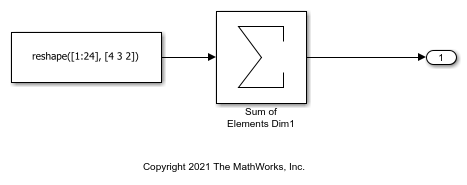Row-Major Algorithms for Row-Major Array Layout

This example shows how to use row-major algorithms to generate efficient code. You can enable the Use algorithms optimized for row-major array layout configuration parameter to enable efficient row-major algorithms that are optimized for row-major array layout. The code that you generate by using row-major algorithms performs with better speed and efficient memory usage when operating on data with row-major array layout.

In this example, you operate on row-major data by first using the default column-major algorithms, and then using the row-major algorithms. This comparison helps in identifying the appropriate algorithm settings to achieve different requirements.

Generate Code by Using the Default Column-Major Algorithms for Row-Major Array Layout

Open the example model ex_rowmajor_algorithm.

model = 'ex_rowmajor_algorithm';
open_system(model);The model contains a Sum of Elements block and the input of the block is an array. By default, Simulink configures a model with column-major algorithms and column-major array layout. In this example, you configure the array layout of this model as row-major. To specify the array layout, open the Embedded Coder app, and then open the Configuration Parameters dialog box. On the Code Generation > Interface pane, set the configuration parameter Array layout to Row-Major option. Alternatively, in the MATLAB Command Window, enter:

set_param(model,'ArrayLayout','Row-major');

Change your current folder in MATLAB to a writable folder. Then generate code from the model by using the slbuild function or by pressing Ctrl+B.

currentDir = pwd;
[~,cgDir] = rtwdemodir();
% Generate code and capture the code generation information to Value
Value=evalc('slbuild(''ex_rowmajor_algorithm'')');

Inspect the generated ex_rowmajor_algorithm_step step function in the ex_rowmajor_algorithm.c.

file = fullfile('ex_rowmajor_algorithm_ert_rtw','ex_rowmajor_algorithm.c');
rtwdemodbtype(file,'/* Model step function */','/* Model initialize function',1,1);
/* Model step function */
void ex_rowmajor_algorithm_step(void)
{
int32_T i;
int32_T i_0;

/* Sum: '<Root>/Sum of Elements Dim1' incorporates:
*  Constant: '<Root>/Constant1'
*/
ex_rowmajor_algorithm_Y.Out2 = -0.0F;
for (i = 0; i < 2; i++) {
for (i_0 = 0; i_0 < 3; i_0++) {
int32_T tmp;
tmp = (i_0 << 1) + i;
ex_rowmajor_algorithm_Y.Out2 =
(((ex_rowmajor_algorithm_ConstP.Constant1_Value[tmp] +
ex_rowmajor_algorithm_Y.Out2) +
ex_rowmajor_algorithm_ConstP.Constant1_Value[tmp + 6]) +
ex_rowmajor_algorithm_ConstP.Constant1_Value[tmp + 12]) +
ex_rowmajor_algorithm_ConstP.Constant1_Value[tmp + 18];
}
}

/* End of Sum: '<Root>/Sum of Elements Dim1' */
}

When Array layout is set to Row-major and the Use algorithms optimized for row-major array layout configuration parameter is set to off, the code generator uses column-major algorithms. The algorithms traverse the data in column-major order though the data is in row-major order. This process requires some extra operations in the generated code, which makes the code less efficient but at the same time results in higher accuracy of numeric results. If you need higher accuracy of numeric results where less efficient code is not a concern, it is recommended that you use the column-major algorithms for row-major array layout.

Generate Code by Using the Row-Major Algorithms for Row-Major Array Layout

To enable the row-major algorithms, on the Math & Data Types pane, select the configuration parameter Use algorithms optimized for row-major array layout check box. This parameter enables the algorithms that are optimized for row-major array layout. Alternatively, in the MATLAB Command Window, enter:

set_param(model,'UseRowMajorAlgorithm','on');

After enabling the row-major algorithms, generate code.

Value=evalc('slbuild(''ex_rowmajor_algorithm'')');

Inspect the generated ex_rowmajor_algorithm_step step function in the ex_rowmajor_algorithm.c.

file = fullfile('ex_rowmajor_algorithm_ert_rtw','ex_rowmajor_algorithm.c');
rtwdemodbtype(file,'/* Model step function */','/* Model initialize function',1,1);
/* Model step function */
void ex_rowmajor_algorithm_step(void)
{
int32_T i;

/* Sum: '<Root>/Sum of Elements Dim1' incorporates:
*  Constant: '<Root>/Constant1'
*/
ex_rowmajor_algorithm_Y.Out2 = -0.0F;
for (i = 0; i < 24; i++) {
ex_rowmajor_algorithm_Y.Out2 +=
ex_rowmajor_algorithm_ConstP.Constant1_Value[i];
}

/* End of Sum: '<Root>/Sum of Elements Dim1' */
}

In comparison to the previous code, when the Array layout is set to Row-major and the Use algorithms optimized for row-major array layout configuration parameter is set to on, the code generator generates efficient code. The code generator uses row-major algorithms that traverse the data in row-major order, which reduces the number of operations in the generated code. But the accuracy of numeric results might not be as high as the previous example. So, you might experience minor numeric differences in the output of column-major and row-major algorithms. If you need an efficient code where numeric accuracy is not a concern, it is recommended that you use the row-major algorithms for row-major array layout.